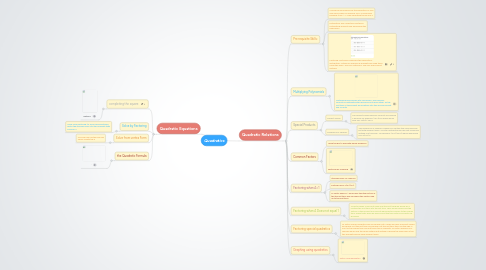Get Started. It's FreeQuadratics### 1.1. Pre requisite Skills:

1.1.1. Classifying Polynomials by the separation of plus and minus signs for example: 2x+3 is a binomial because it has 1 "+" sign separating the 2x and 3.

1.1.2. Distribution and Collecting Like terms: Distributing brackets and simplifying the expression.

1.1.3. Factoring: Factoring is basically the opposite of distribution. Instead of opening up brackets we make them. using two ways. The GCF method or The sum and product method.

### 1.2. Multiplying Polynomials

1.2.1. Multiplying polynomials into monomials: This basically consists of distributing two binomials into each other. As the first term in the bracket will mupltiply into the second bracket and so forth.

### 1.3. Special Products

1.3.1. Perfect square:

1.3.1.1. The Perfect square basically consists of squaring a binomial for example. (2x+3)Sq. which would give you 4x(sq)+12x+9

1.3.2. Difference of squares:

1.3.2.1. The Difference of squares is when you multiply the same binomial but with different signs. So after distributing you will get a binomial instead of a trinomial. For example: (2x-3)(2x+3) which would give you 4x(Sq)-9

### 1.4. Common Factors

1.4.1. Using the gcf to eliminate harder problems.

1.4.2. Factoring by Grouping

### 1.5. Factoring when A=1

1.5.1. Standard form: a2+2ab+b2

1.5.2. Factored form: a(x-r)(x-s)

1.5.3. To factor when a=1 we usually take the factors of the the last term and see which two factors add up to the first term.

### 1.6. Factoring when A Does not equal 1

1.6.1. To factor when a does not equal one the first thing we would do is multiply the first term into the last term. Then we we would find the factors of the product and look at which factors add up to the middle term. When thats done we would input the two factors and factor by grouping.

1.7.1. To factor special quadratics we use square roots. When we have a perfect square we square root the first term and square root the last term then use the sign in front of the middle term and put those two in brackets. To factor difference of squares we will use the same method but instead of having the same sign in the two brackets we will have different signs.

2.1.1. method:

### 2.2. Solve by Factoring

2.2.1. Solve using factoring: to solve using factoring, factor the standard form into two brackets then solve for x.

### 2.3. Solve from vertex Form

2.3.1. To solve from vertex form we simply isolate the x.